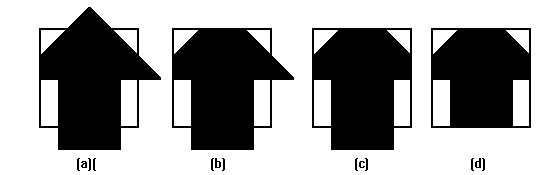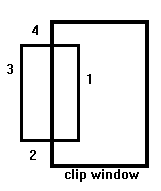# Sutherland - Hodgman Polygon Clipping

The Sutherland - Hodgman algorithm performs a clipping of a polygon against each window edge in turn. It accepts an ordered sequence of verices v1, v2, v3, ..., vn and puts out a set of vertices defining the clipped polygon.This figure represents a polygon (the large, solid, upward pointing arrow) before clipping has occurred.

The following figures show how this algorithm works at each edge, clipping the polygon.1. Clipping against the left side of the clip window.

2. Clipping against the top side of the clip window.

3. Clipping against the right side of the clip window.

4. Clipping against the bottom side of the clip window.

## Four Types of Edges

As the algorithm goes around the edges of the window, clipping the polygon, it encounters four types of edges. All four edge types are illustrated by the polygon in the following figure. For each edge type, zero, one, or two vertices are added to the output list of vertices that define the clipped polygon.The four types of edges are:

1. Edges that are totally inside the clip window. - add the second inside vertex point
2. Edges that are leaving the clip window. - add the intersection point as a vertex
3. Edges that are entirely outside the clip window. - add nothing to the vertex output list
4. Edges that are entering the clip window. - save the intersection and inside points as vertices

## How To Calculate Intersections

Assume that we're clipping a polgon's edge with vertices at (x1,y1) and (x2,y2) against a clip window with vertices at (xmin, ymin) and (xmax,ymax).

The location (IX, IY) of the intersection of the edge with the left side of the window is:

1. IX = xmin
2. IY = slope*(xmin-x1) + y1, where the slope = (y2-y1)/(x2-x1)

The location of the intersection of the edge with the right side of the window is:

1. IX = xmax
2. IY = slope*(xmax-x1) + y1, where the slope = (y2-y1)/(x2-x1)

The intersection of the polygon's edge with the top side of the window is:

1. IX = x1 + (ymax - y1) / slope
2. IY = ymax

Finally, the intersection of the edge with the bottom side of the window is:

1. IX = x1 + (ymin - y1) / slope
2. IY = ymin

## Some Problems With This Algorithm

1. This algorithm does not work if the clip window is not convex.
2. If the polygon is not also convex, there may be some dangling edges.

From P. Asokarathinam -- see details 27.11.1996